• 如何使用python画曲线图？下面是基本步骤：前提首先，为了实际使用 Matplotlib，我们需要安装它。安装如果你安装了更高版本的 Python，你应该能够打开cmd.exe或终端，然后执行：pip install matplotlib注意：如果...如何使用python画曲线图？下面是基本步骤：
前提
首先，为了实际使用 Matplotlib，我们需要安装它。
安装
如果你安装了更高版本的 Python，你应该能够打开cmd.exe或终端，然后执行：pip install matplotlib
注意：如果上面的较短命令不工作，你可能需要执行C:/Python34/Scripts/pip install matplotlib。
如果在导入matplotlib时，你会收到类似『无命名模块』和模块名称的错误，这意味着你还需要安装该模块。 一个常见的问题是缺少名为six的模块。 这意味着你需要使用pip安装six。
或者，你可以前往 Matplotlib.org 并通过访问下载页面下载适当的版本进行安装。请记住，因为你的操作系统为 64 位，你不一定需要 64 位版本的 Python。 如果你不打算尝试 64 位，你可以使用 32 位。 打开 IDLE 并阅读顶部。 如果它说你是 64 位，你就是 64 位，如果它说是 32 位，那么你就是 32 位。 一旦你安装了 Python，你就做好了准备，你可以编写任何你想要的逻辑。我喜欢使用 IDLE 来编程，但你可以随意使用任何你喜欢的东西。
第一步：
打开Python的shell界面，如图所示：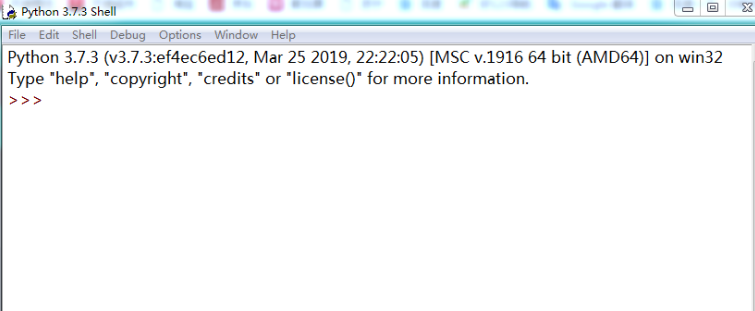第二步：
输入以下代码导入我们用到的函数库。>>> import numpy as np
>>> import matplotlib.pyplot as plt
第三步：
产生我们要画的的函数的数据，以sin函数为例，代码如下：>>> x=np.arange(0,5,0.1);
>>> y=np.sin(x);
第四步：
输入以下指令我们就可以看到我们要画的函数了。
plt.plot(x,y)
第五步：
我们可以查看到，我们要画的图像了。
采用刚才代码后有可能无法显示下图，然后在输入以下代码就可以了：
plt.show()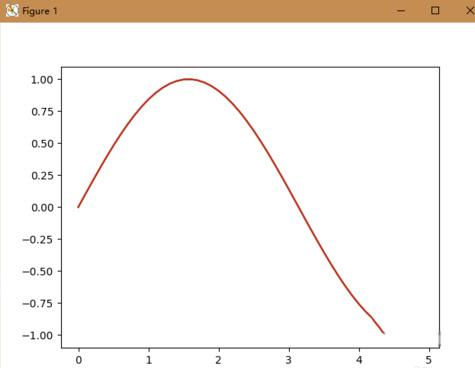展开全文• python plot画简单的曲线图 ubuntu jupyter notebook 简单的曲线图，但显示中文时有问题，以下画曲线图指令和中文显示解决方法： 1.简单代码 import matplotlib.pplot as plt from pylab import * plt.rcParams['...
python plot画简单的曲线图
ubuntu jupyter notebook 画简单的曲线图，但显示中文时有问题，以下画曲线图指令和中文显示解决方法： 1.简单代码
import matplotlib.pplot as plt
from pylab import *
plt.rcParams['font.sans-serif']=['SimHei'] #用来正常显示中文标签
pic1 = plt.subplot(2,1,1 )#画多个图用到 （行，列，活跃区）
plt.plot(x_data,y_data,'y*-')   #'y*-'指定颜色和线条样式

pic2 = plt.subplot(2,2,2 )#与pic1共享y周）
plt.plot(x_data,y_data,'y*-',linewidth=4，label=‘线条示例注释’)
plt.xlabel("x轴的名称"，fontsize=10）
plt.ylabel（"y轴的名称"，fontsize=10）
plt.title（“标题名称”，fontsize）
plt.legend(loc = 'upper right',bbox_to_anchor = (1,1) #线条示例框放什么位置
plt.show

2,.解决中文不显示办法 直接参考博客https://blog.csdn.net/sunmingyang1987/article/details/102835614 其他画图参考
展开全文• ## python画蝴蝶曲线图

万次阅读 2018-11-07 16:40:40
由于此曲线优美，因此就想把它作为博客favicon.ico，这里我使用pytho matplotlib.pyplot包来绘制需要的蝴蝶曲线图。 先看下漂亮的蝴蝶曲线吧。 #1.首先我们需要确定蝴蝶曲线的函数表达 也可用球坐标表示 #2....
蝴蝶曲线是由Temple H·Fay发现的可用极坐标函数表示的蝴蝶曲线。 由于此曲线优美， 因此就想把它作为博客favicon.ico，这里我使用pytho matplotlib.pyplot包来绘制需要的蝴蝶曲线图。 先看下漂亮的蝴蝶曲线吧。1.首先我们需要确定蝴蝶曲线的函数表达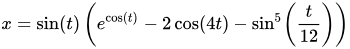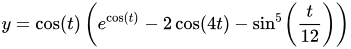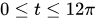也可用球坐标表示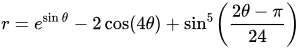2.选择python里面的matplotlib.pyplot作为画图工具
1.首先导入python包
import numpy as np
import matplotlib.pyplot as plt

2.设置个参数的值
t = np.arange(0.0, 12*np.pi, 0.01)
x = np.sin(t)*(np.e**np.cos(t) - 2*np.cos(4*t)-np.sin(t/12)**5)
y = np.cos(t)*(np.e**np.cos(t) - 2*np.cos(4*t)-np.sin(t/12)**5)

3.根据公式，使用numpy里面的函数使用plt画出所需图片
plt.figure(figsize=(8,6))
plt.axis('off')
plt.plot(x,y,color='blue',linewidth = '2')
#plt.show()
plt.savefig("butter.jpg",dpi=400)4.使用Image重新调整图片到适当的大小使其符合favicon大小
from PIL import Image
im = Image.open("butter.jpg")
favicon = im.resize((50,50))
favicon.save("favicon.ico")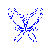至此，我们完成了使用python的matplotlib.pyplot包绘制漂亮的蝴蝶曲线的过程，把图片保存到网站跟目录就可以看到了！ 个人博客本文地址：https://www.bobobk.com/24.html
展开全文• 如何使用python画曲线图?下面是基本步骤：前提首先，为了实际使用 Matplotlib，我们需要安装它。安装如果你安装了更高版本的 Python，你应该能够打开cmd.exe或终端，然后执行：pip install matplotlib注意：如果上面...如何使用python画曲线图?下面是基本步骤：
前提
首先，为了实际使用 Matplotlib，我们需要安装它。
安装
如果你安装了更高版本的 Python，你应该能够打开cmd.exe或终端，然后执行：pip install matplotlib
注意：如果上面的较短命令不工作，你可能需要执行C:/Python34/Scripts/pip install matplotlib。
如果在导入matplotlib时，你会收到类似『无命名模块』和模块名称的错误，这意味着你还需要安装该模块。 一个常见的问题是缺少名为six的模块。 这意味着你需要使用pip安装six。
或者，你可以前往 Matplotlib.org 并通过访问下载页面下载适当的版本进行安装。 请记住，因为你的操作系统为 64 位，你不一定需要 64 位版本的 Python。 如果你不打算尝试 64 位，你可以使用 32 位。 打开 IDLE 并阅读顶部。 如果它说你是 64 位，你就是 64 位，如果它说是 32 位，那么你就是 32 位。 一旦你安装了 Python，你就做好了准备，你可以编写任何你想要的逻辑。 我喜欢使用 IDLE 来编程，但你可以随意使用任何你喜欢的东西。
第一步：
打开Python的shell界面，如图所示。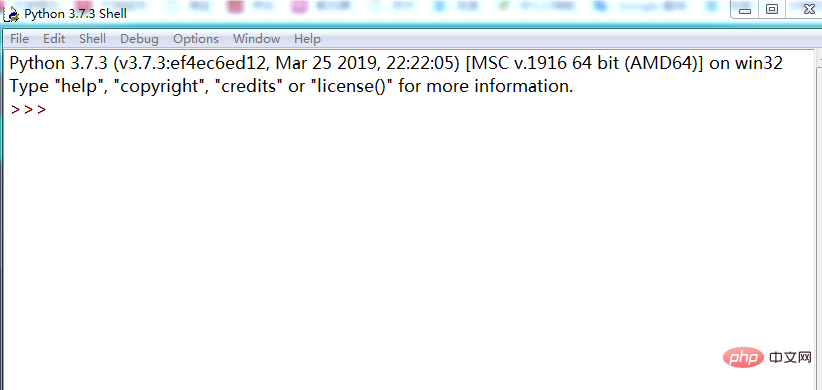第二步：
输入以下代码导入我们用到的函数库。>>> import numpy as np
>>> import matplotlib.pyplot as plt
第三步：
产生我们要画的的函数的数据，以sin函数为例，代码如下。>>> x=np.arange(0,5,0.1);
>>> y=np.sin(x);
第四步：
输入以下指令我们就可以看到我们要画的函数了。plt.plot(x,y)
第五步：
我们可以查看到，我们要画的图像了。
采用刚才代码后有可能无法显示下图，然后在输入以下代码就可以了：
plt.show()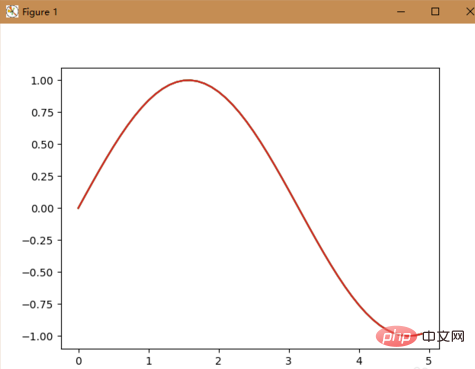以上就是如何使用python画曲线图的详细内容，更多请关注php中文网其它相关文章！
本文原创发布php中文网，转载请注明出处，感谢您的尊重！
展开全文• Python如何函数的曲线输入以下代码导入小编们用到的函数库。 >>> import numpy as np >>> import matplotlib.pyplot as plt >>> x=np.arange(0,5,0.1); >>> y=np.sin(x); plt.plot(x,y) 采用刚才代码后有可能无法...
• import numpy as np import pandas as pd import matplotlib.pyplot as plt plt.figure(1) # 创建图表1 plt.figure(2) # 创建图表2 ax1 ...y[x] = 0 y[x>169]=1000 plt.plot(x, y) plt.show() 使用Python画数学函数曲线
• python画二维曲线图plot import numpy as np import pylab as pl times=np.arange(0,5,0.01) #times为x的值，0为起点，5为终点，0,01为步长 fun=lambda x:np.cos(20*x)*np.exp(-pl.absolute(x)) #fun为关于x的函数...
• plt.xlabel('Date') plt.ylabel('Number') plt.show() 到此这篇关于利用python绘制数据曲线图的实现的文章就介绍到这了,更多相关python 数据曲线图内容请搜索脚本之家以前的文章或继续浏览下面的相关文章希望大家...
• python画线 import matplotlib.pyplot as plt x = (155,159,164,168,172) y = (48,50,53,56,60) plt.plot(x,y,alpha=0.6) plt.show python画点图 import matplotlib.pyplot as plt x = (155,159,164,168,172) y ...
• 浏览： 次 日期：2018年9月2日【下载文档: python matlibplot绘制多条曲线图.txt 】(友情提示:右键点上行txt文档名->目标另存为)python matlibplot绘制多条曲线图 这里我利用的是matplotlib.pyplot.plot的工具来绘制...
• python画折线利用的是matplotlib.pyplot.plot的工具来绘制折线，这里先给出一个段代码和结果：# -*- coding: UTF-8 -*-import numpy as npimport matplotlib as mplimport matplotlib.pyplot as plt#这里导入...
• 这篇文章利用的是matplotlib.pyplot.plot的工具来绘制折线，这里先给出一个段代码和结果：# -*- coding: UTF-8 -*-import numpy as npimport matplotlib as mplimport matplotlib.pyplot as plt#这里导入你自己...
• 1. 首先导入一些python画图的包，读取txt文件，假设我现在有两个模型训练结果的records.txt文件import numpy as npimport matplotlib.pyplot as pltimport pylab as plfrom mpl_toolkits.axes_grid1.inset_locator ...
• ## 如何通过python画loss曲线

万次阅读 多人点赞 2019-01-23 14:53:38
如何通过python画loss，PSNR, SSIM曲线 首先导入一些python画图的包，读取txt文件，假设我现在有两个模型训练结果的records.txt文件 import numpy as np import matplotlib.pyplot as plt import pylab as ...
• 正常的使用plot绘制图像时，边缘都会存在空白边框，保存的结果可能不满足需求，所以想要去除周围的空白边框。之前查了一些资料，发现找到的方法上仍存在不足，之后尝试并总结了一下代码，成功地实现了该功能。代码...
• ## python画正弦曲线

千次阅读 2019-09-29 02:24:21
正弦曲线先设定一下x的取值范围，从0到2π。要用到numpy模块。 numpy.pi 表示π numpy.arange( 0 , 2π ,0.01) 从0到2π，以0.01步进。 令 x=numpy.arange( 0, 2*numpy.pi, 0.01) y=numpy.sin(x) 画图要...
•   这里我利用的是matplotlib.pyplot.plot的工具来绘制折线，这里先给出一个段代码和结果： # -*- coding: UTF-8 -*- import numpy as np import matplotlib as mpl import matplotlib.pyplot as plt #这里...
• ## python画log曲线

千次阅读 2020-01-08 19:18:53
import matplotlib.pyplot as plt import numpy as np x = np.arange(0, 3, 0.01) y = np.log(x)/10 plt.title("一元一次函数") plt.plot(x, y) plt.show()
• 以下是整理封装好的曲线图画法： import matplotlib . pyplot as plt import numpy as np from scipy . interpolate import spline import json , time def test ( ) : ###################...
• 2.3.2例子二次函数绘制方法把二次函数图像变成点存储于list中直线的时候我们了三个点，我们还可以更多的点。点越多图像就越是一条直线，运用的思想是微积分的思想。如何生成很多点呢?python本身自带range()...
• 一、使用Matplotlib生成数据：是Python上的一个2D绘图库，它可以在跨平台上边出很多高质量的图像。1、Pycharm安装Matplotlib库(1)点击菜单上的"file” -> "settings”：(2)选中你的项目（比如thisyan ...
• 到了折线分析的时候，在想用哪些工具的时候。首先否决了excel，读书人的事，怎么能用excel论文的呢？ 然后我又尝试了Gnuplot、Matlab、Python等。这些软件作图无疑是一个非常好的选择，他们都有一个共同的...可视化 uboot matlab
• python画新型冠状病毒病例曲线图 地图的，因为要下载地图工具包，所以没去深入学习。 #!/usr/bin/env python3 # -*- coding: utf-8 -*- """ 功能：用python画新型冠状病毒病例曲线图 环境：python3.7 日期...
• 今天小老婆给我抱怨说复习得很累，写了特别多的东西。...然后我感觉以我水平应该可以用python画一下这个。 说干就干。 import matplotlib.pyplot as plt import numpy as np from pylab impor...
• 事实上在很多时候，例如写论文，例如写报告，例如做ppt，都需要花很多很多曲线图，让人家信服 毕竟数据可视化是人的本能。 假如读者您很不幸，像我一样不会用matlab之类的东西画图或者没办法用matlab画图，那么可以......

# python画plot曲线图python 订阅